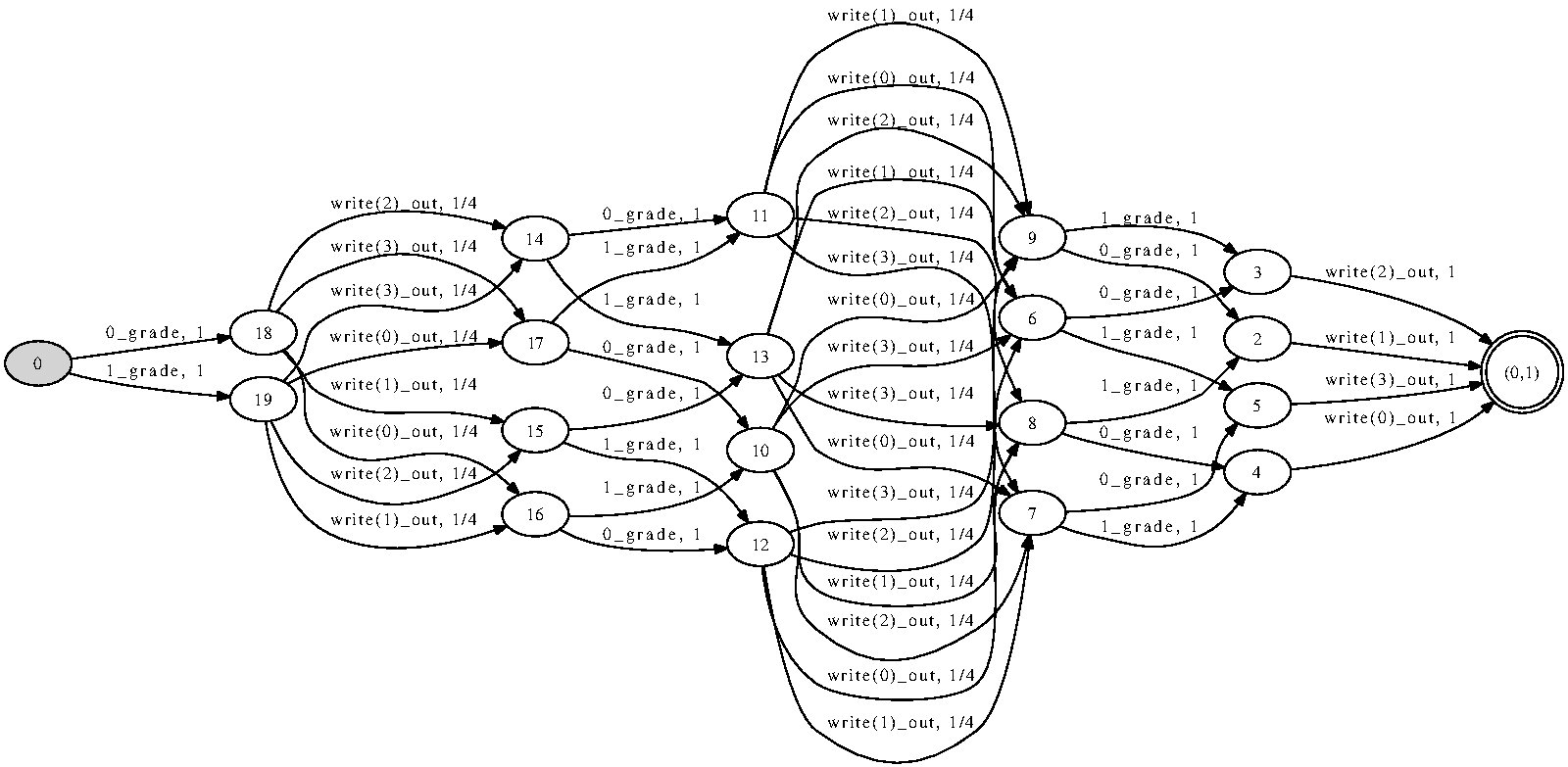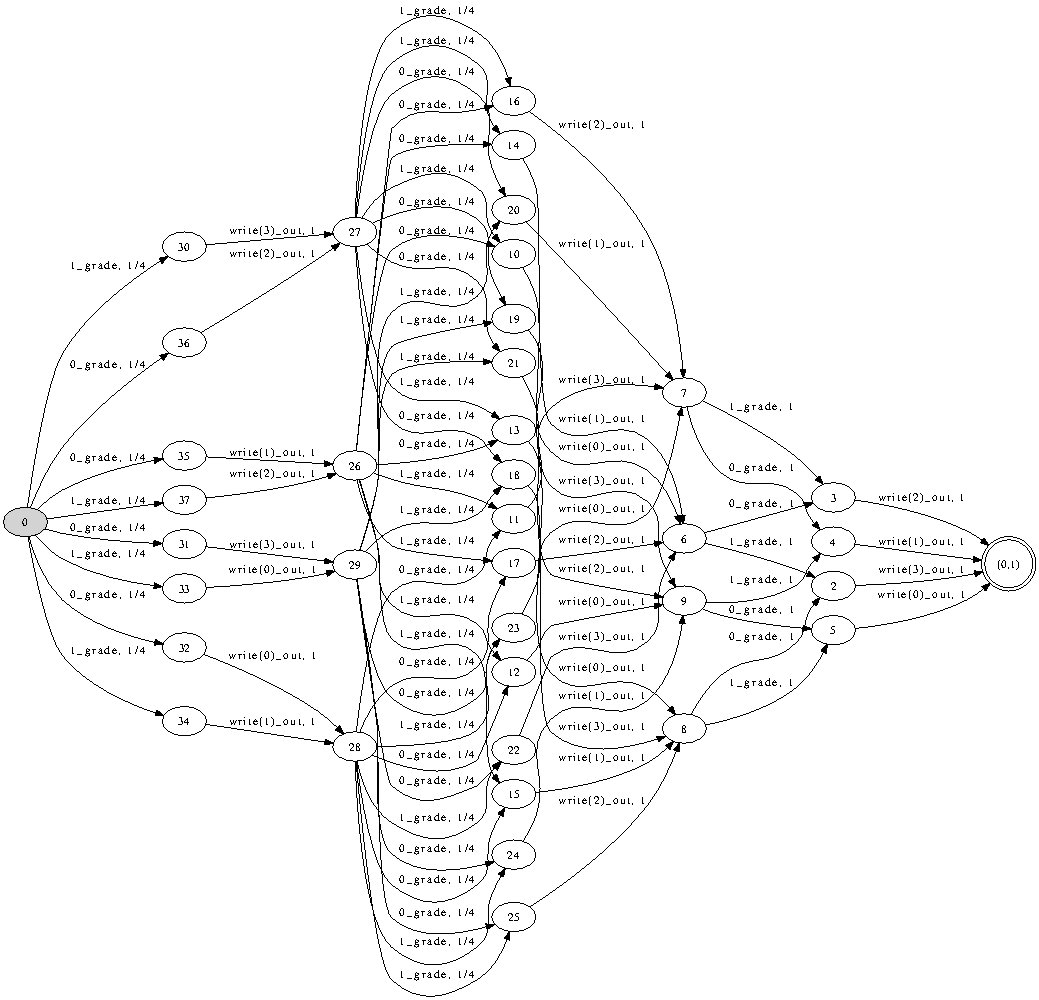Try it with APEX!

## Background

Assume that a group of students has been graded and would like to find out the sum of all their grades, e.g. to compute the average. However, none wants to reveal their individual grade in the process. The task can be accomplished with the following randomised algorithm. Let S ∈ N be the number of students, and let {0, · · · , G − 1} (G ∈ N) be the set of grades. Define N = (G − 1) · S + 1. Further we assume that the students are arranged in a ring and that each pair of adjacent students shares a random integer between 0 and N − 1. Thus a student shares a number l with the student on the left and a number r with the student on the right, respectively. Denoting the student’s grade by g, the student announces the number (g + l − r) mod N . Because of the ring structure, each number will be reported twice, once as l and once as r, so the sum of all announcements (modulo N ) will be the same as the sum of all grades.

What we can verify is that only this sum can be gleaned from the announcements by a casual observer, i.e. the observer cannot gain access to any extra information about individual grades. This correctness condition can be formalised by a specification, in which the students make random announcements subject to the condition that their sum equals the sum of their grades. Correct protocol. To check correctness of the Grade protocol, we check if the automaton resulting from the implementation of the protocol is equivalent to the specification automaton. Both automata are presented in Figure 5 for G = 2 and S = 3. Both automata accept words which are sequences of grades and announcements. The words are accepted with the same probability as that of producing the announcements for the given grades. Adherence to specification can then be verified via language equivalence. In Figure 6 we report various data related to the performance of apex and the equivalence checking algorithms. The reported times tspec and tprot are those needed to construct the automata corresponding to specification and the pro- tocol respectively (we also give their sizes sspec , sprot ). The columns labelled by t←,rand , t←,det, t→,rand, t→,det give the running time of the language equivalence check of the respective automata using the four algorithms. The symbol − means that the computation timed out after 10 minutes. The running time of the deterministic backwards algorithm is higher than the one of the forward algorithm because the backwards algorithm constructs a different vector space with a higher number of dimensions.
```// PRINTS OUT PAIRS (GRADE,ANNOUNCEMENT)
// ANONYMITY CAN BE VERIFIED BY CHECKING
// LANGUAGE EQUIVALENCE WITH SPECIFICATION (grade-spec.txt)

// number of students
//const STUDENTS:= 3;

var%(STUDENTS+1) i;

var%N first;
first:=rand[N];

var%N right;
right:=first;

i:=0;
while(i<STUDENTS) do {
var%N left;
i:=succ(i);
left :=  if (i=STUDENTS) then first else rand[N];
out := (grade + left) - right;
right := left;
}
:com
```

```// SPECIFICATION TO BE USED WITH grade-impl2.txt

//const STUDENTS:=10;

const N := STUDENTS * (GRADES-1) + 1;

var%STUDENTS i;
var%N total;

i:=1;
while(i) do {
var%N r;
r := rand[N];
out:=r;
total := total - r;
i:=succ(i)
};